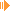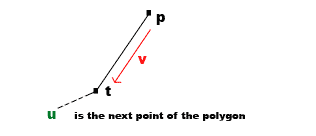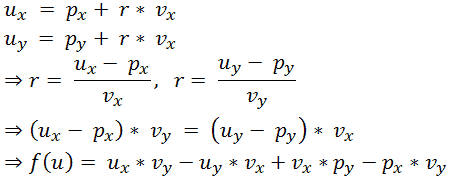Polygon ConvexityDownload Applet with Source (18kb)

After searching for a simple algorithm for checking if a (simple!) polygon is convex, I came across a quite simple but interesting algorithm. (A simple polygon does not contain any holes and the boundary of the polygon does not cross itself). First two easy definitions:

 Convex Set: A set P is convex if for every two points p1, p2 within this set, the straight line between them is also completely within this set.

 Convex Polygon: A simple polygon P is convex if its interior is a convex set.

For example:Ok, so to check the convexity of a polygon, you can think about it like this: If you move along the sides of the polygon and you just have to move to the left or the right, then it is convex. If you have to change the direction at least once, then it is not convex. In this way it doesn't matter if you walk along the sides in clockwise or counterclockwise direction.

Sounds pretty obvious but how to find out if the next point is right or left from the current polygon edge? Have a look at following image: p and t are two consecutive vertices of the polygon.The line g is the line through them, we can write it in a form like this:So you can calculate all points u on the line g by inserting any r values you like. Let's eliminate r...The function f has following properties: f(u) = 0 if the next polygon vertice u is on the line; f(u) > 0 if the next polygon vertice u is right from the line g and f(u) < 0 if the next polygon vertice u is left from the line g. "Left" and "Right" depends on the direction of vector v, so it depends on going along the polygon clockwise or counterclockwise.
Next applet shows you exactly this for three points. You can play around a bit :-)

So to check a complete polygon is very easy - just walk along all vertices and check if the direction changes. This is implemented in the applet belonging to the article about filling arbitrary polygons on my site, but here is the source:

 /*  * Variable polygon is a vector of points.  * Returns true if polygon is convex, otherwise false.  */ public boolean checkConvexity() {   if (polygon.size() < 3) return false;   Point p;   Point v;   Point u;   int res = 0;   for (int i = 0; i < polygon.size(); i++)   {     p = polygon.get(i);     Point tmp = polygon.get((i+1) % polygon.size());     v = new Point();     v.x = tmp.x - p.x;     v.y = tmp.y - p.y;     u = polygon.get((i+2) % polygon.size());     if (i == 0) // in first loop direction is unknown, so save it in res       res = u.x * v.y - u.y * v.x + v.x * p.y - v.y * p.x;     else     {       int newres = u.x * v.y - u.y * v.x + v.x * p.y - v.y * p.x;       if ( (newres > 0 && res < 0) || (newres < 0 && res > 0) )         return false;     }   }   return true; }

This site is part of Sunshine's Homepage